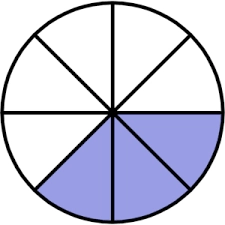# Evaluate expression 10

Evaluate expression: (a-b)+c if a=7/10, b=3/5, and c=-1.9. Write your answer as a mixed number in simplest form.

x =  -9/5

### Step-by-step explanation:Did you find an error or inaccuracy? Feel free to write us. Thank you!

Tips for related online calculators
Need help calculating sum, simplifying, or multiplying fractions? Try our fraction calculator.